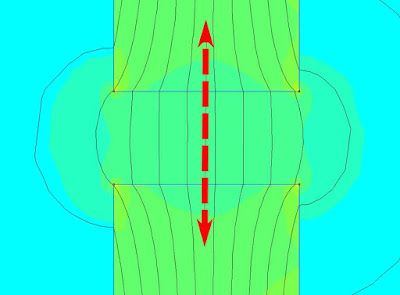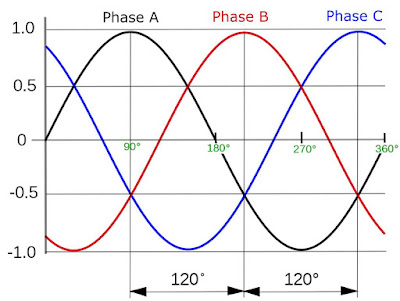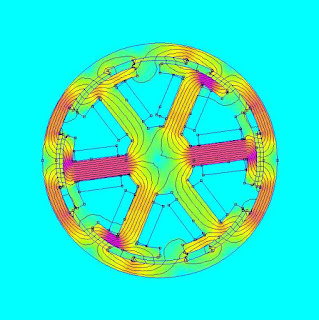## Wednesday, December 26, 2018

### Understanding BLDC (PMSM) electric motor constants - Optimal flux gap for high torque density

For many weight sensitive applications in robotics, it is desirable to have high torque density actuators. It is also often desirable to have relatively low gear ratios as this improves un-sensored output torque accuracy and helps to minimise rotational inertia, which improves angular acceleration. This is the basis of Ben Katz low-cost modular actuator design. There is, therefore, a need for electric motors with as high a gravimetric torque density (torque per unit mass) as possible.

Despite this, I have so far been unable to find much information online in the 'hobbyist community' (i.e. non-academic) about which aspects of an electric motor are important for torque density and how they can be optimised. Therefore, for the next series of posts I will be using FEMM and a simple motor model to try and develop a working understanding of how different motor parameters (e.g. flux gap size, magnet length, stator tooth shape, slot and pole number etc.) impact the torque density of a brushless permanent magnet synchronous motor.

Let's get started.

## The flux gap

The flux gap is the distance between the high magnetic permeability material in the stator (stator 'iron') and the corresponding high magnetic permeability material in the rotor (rotor 'back iron'). This material is normally made of thin laminations of Fe-Si steel.

It is well known that, in general, the flux gap should be as small as possible.

It is important to note here that the flux gap includes the magnets. Rare earth magnets (magnetised or un-magnetised) have a magnetic permeability essentially the same as air. Therefore, from the stators perspective, a magnet is no different than air and should be included as part of the flux gap.

Let's start by considering two simple magnetic circuits simulated in FEMM; one with a 1 mm flux gap and one with a 4 mm flux gap.1 mm flux gap core4 mm flux gap
The magnetic circuit consists of a ring of soft iron (literally pure annealed iron), copper windings represented by the green rectangles (2A, 250 turns) and an air gap in the ring. The flux density (unit of Tesla) is represented by how close the lines of flux are together and by the colour, with red being the highest density and blue being the lowest. The flux density in the ring with the small flux gap is clearly the largest. This ring also has the least amount of flux 'leaking' out into the surrounding air.

The reason for this difference is that an air gap increases the magnetic reluctance of the circuit. Magnetic reluctance is to flux in a magnetic circuit what resistance is to a current in an electric circuit. Therefore, the magnetic flux in the circuit is dependent upon the total magnetic reluctance and the applied magneto-motive force (turns times current) just as an electrical current is dependent upon the total resistance in an electric circuit and the applied voltage. For a nice overview of the concept check out Ben Krasnow's video on the topic.

Let's look more closely at how the flux changes over the flux gap itself. We can do this by drawing a line over the flux gap and measuring the flux density at each point on the line.The 4 mm flux gap and line which we will measure flux density
Doing this for both the 1 mm and 4 mm flux gap it is clear that the flux in the middle of each gap remains constant. It can also be seen that the flux is four times smaller in the 4 mm flux gap than the 1 mm flux gap. Therefore, in order to produce the same flux density in the 4 mm gap, we would need to either add four times as many windings at the same current or alternatively, keep the same number of windings and add four times as much current. This concept can also be applied to electric motors and explains why engineers generally do everything they can to keep the flux gap as small as possible.

The flux density in the flux gap can be approximated using the following equation:

B=\frac{\mu_{0}NI}{g}

where B is the flux density (Tesla), mu_{0} is the magnetic permeability of free space (4\pi\times10^{-7}), N is the number of turns of wire, I is the current (Ampere) and g is the flux gap (meters). Plotting B vs g we see the following:

This equation assumes that the reluctance of the iron core is negligible. This is a safe assumption in this example as reluctance of the Fe core is around 10^{-7} times smaller than the reluctance of the flux gap and so can be disregarded. However, if your core is close to saturation, as would be the case if you reduce the flux gap to zero, then this will not always be the case. Also, this equation can only be used for a constant cross-section like that of the 'racetrack' core shown above, but does provide a good starting point for cores of other shapes. For best results a FEA package like FEMM (its free!) will give the best approximation.

Unlike the simple magnetic circuits shown above, the flux gap problem is made more complicated for BLDC motors for a few reasons:
1. There are multiple flux gaps. The stator flux must travel across to the rotor and back again and can do so at multiple points.
2. The magnetic permeability, and hence the magnetic reluctance, of the ferromagnetic stator and rotor back iron, is not constant but instead depends on the total amount of flux in that region.
Note that simply embedding the magnets in the rotor back iron does not eliminate the flux gap, it only moves it further back into the rotor. Embedded rotor magnets do have their own advantages (improved field weakening performance, control over reluctance torque) but they are topics for another day.

## Effect of flux gap size on torque for a simple motor

In order to explore the impact of the flux gap size on something more closely resembling a real  motor I have simulated a 6 slot, 8 pole 'out runner'. The motor was sketched in F360 and exported as a dxf file for use in FEMM. It has a stator diameter of 57 mm and a rotor length (into screen) of 10 mm. This motor has three phases which are wound with concentrated windings (double layered) as ABCABC. A current of 50A is supplied on phase A, and -25A is supplied on phase B and C so that all of the flux is directed on the Q-axis where it will produce the most torque.The motor is simulated with a fixed current at 90 degree
Shane Colton's blog post on field orientation has a good rundown on the q-axis and d-axis argument.  In short, the phase with the most current on it (phase A) is 90 electrical degrees ahead (q-axis) of the direct flux of the magnets (d-axis) where it will produce the most torque per amp. If the rotor was rotating then so too would the magnetic field generated in the stator so that the torque remains constant and proportional to the current.Flux density due to the stator windings only (magnets removed)
However, in this simulation, both the current and rotor position are fixed and we are instead only solving for the flux density generated in the air gap by the stator windings and the magnets.
In the simulation seen below the flux is seen to be concentrated in the high magnetic permeability stator teeth and in the back iron of the rotor. This flux almost exclusively crosses at the flux gap.1 mm flux gap
For comparison, a motor with a 3mm flux gap is shown below. Here we can see that the total flux density in the stator teeth and back iron is greatly reduced due to the increased magnetic reluctance in the magnetic circuit. This decrease is also seen in the flux gap where the magnets are located.3 mm flux gap
If you would like to play around with these models in FEMM you can find the files hosted here. This tutorial gives you enough information to get started if you have never used FEMM before.

Using FEMM it is also possible to estimate the static torque that this 10 mm long rotor would produce. The torque and flux density within the flux gap with respect to the flux gap size is shown below.

We can see two things quite clearly: i) the torque produced by the motor is dependent upon the flux density in the air gap and ii) the torque falls off asymptotically as the flux gap size is increased. Note that in this example we are increasing the diameter of the rotor to increase the flux gap. Since motor torque increases with the square of the flux gap diameter the fall off in torque would be much steeper if we were to instead decrease the size of the stator to increase the flux gap.

From the above discussion it is clear that, in general, we want to have as small a flux gap as physically possible so as to increase the motors torque output and, therefore, it's motor constant. However, aside from needing to consider manufacturing tolerances, we also need to consider the thickness of the magnets. In general, if you make the rotor magnets longer then the flux density at their poles is also increased. This will act to increase the torque output of your motor.

The impact of magnet length to motor torque will, therefore, be the topic of the next post.

## Conclusion

Increasing the size of the flux gap for a motor will increase the magnetic reluctance in the magnetic circuit which reduces the flux density in the air gap. The torque generated by a motor is proportional to the flux in the air gap. Therefore, increasing the size of the flux gap will reduce the torque generated by a motor for a fixed winding current, which reduces the overall motor constant.

Equations were produced in this post with the help of arachnoid.com and are based on those found in the book Electric Motors and Drives: Fundamentals, types and applications by Austin Hughes. If you have noticed any errors in the above article then please let me know.